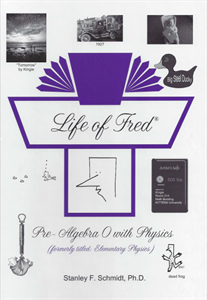# Life of Fred Pre-Algebra 0 with Physics

• \$ 25.00

Fred does physics before algebra.

In Pre-Algebra 0 with Physics you will learn about: Numerals, Making models, Nineteen conversion factor problems, Area of a rectangle, Friction independent of speed, Constants of Proportionality, Exact speed of light, Continuous and discrete variables, Story of the meter, Pendulums, Oblate spheroids, Krypton vs. kryptonite, Square roots, Pilgrims in Massachusetts in 1620, Hard c and soft c, Normal forces, Coefficient of friction, Solving d = rt for r, How ducks pronounce mu, raphing points, Ordered pairs, Why rocks stop falling, Hunch-conjecture-theory-law, Simultaneity doesn’t exist, Inductive and deductive reasoning, How fossils and astronomy are connected, and so much more.

Sample Text